R scripts: hierarchical1.R

Mlxtran codes: model/hierarchical1a.txt ; model/hierarchical1b.txt

$$\newcommand{\esp}{\mathbb{E}\left(#1\right)} \newcommand{\var}{\mbox{Var}\left(#1\right)} \newcommand{\deriv}{\dot{#1}(t)} \newcommand{\prob}{ \mathbb{P}\!(#1)} \newcommand{\eqdef}{\mathop{=}\limits^{\mathrm{def}}} \newcommand{\by}{\boldsymbol{y}} \newcommand{\bc}{\boldsymbol{c}} \newcommand{\bpsi}{\boldsymbol{\psi}} \def\pmacro{\texttt{p}} \def\like{{\cal L}} \def\llike{{\cal LL}} \def\logit{{\rm logit}} \def\probit{{\rm probit}} \def\one{{\rm 1\!I}} \def\iid{\mathop{\sim}_{\rm i.i.d.}} \def\simh0{\mathop{\sim}_{H_0}} \def\df{\texttt{df}} \def\res{e} \def\xomega{x} \newcommand{\argmin}{{\rm arg}\min_{#1}} \newcommand{\argmax}{{\rm arg}\max_{#1}} \newcommand{\Rset}{\mbox{\mathbb{R}}} \def\param{\theta} \def\setparam{\Theta} \def\xnew{x_{\rm new}} \def\fnew{f_{\rm new}} \def\ynew{y_{\rm new}} \def\nnew{n_{\rm new}} \def\enew{e_{\rm new}} \def\Xnew{X_{\rm new}} \def\hfnew{\widehat{\fnew}} \def\degree{m} \def\nbeta{d} \newcommand{\limite}{\mathop{\longrightarrow}\limits_{#1}} \def\ka{k{a}} \def\ska{k{\scriptscriptstyle a}} \def\kel{k{e}} \def\skel{k{\scriptscriptstyle e}} \def\cl{C{\small l}} \def\Tlag{T\hspace{-0.1em}{lag}} \def\sTlag{T\hspace{-0.07em}{\scriptscriptstyle lag}} \def\Tk{T\hspace{-0.1em}{k0}} \def\sTk{T\hspace{-0.07em}{\scriptscriptstyle k0}} \def\thalf{t{1/2}} \newcommand{\Dphi}{\partial_\pphi #1} \def\asigma{a} \def\pphi{\psi} \newcommand{\stheta}{{\theta^\star}} \newcommand{\htheta}{{\widehat{\theta}}}$$

# 1 Introduction

The population approach means that each individual is represented by the same basic parametric model but not necessarily the exact same parameter values. Thus, individual $$i$$ has parameters $$\psi_i$$. If we consider that individuals are randomly selected from the population, then we can treat $$\psi_i$$ as a random vector of parameters.

The probability distribution of $$\psi_i$$ is a parametric distribution that depends on a vector $$\theta$$ of population parameters and possibly a set of individual covariates $$c_i$$. This dependence can be made clear by writing $$\pmacro(\, \psi_i \,;\theta,c_i)$$ for the pdf of $$\psi_i$$.

In this context, the model for individual $$i$$ is the joint distribution of the longitudinal data $$y_i$$ and the individual parameters $$\psi_i$$: $\pmacro(y_i , \psi_i; \theta, c_i)=\pmacro(y_i | \psi_i ) \, \pmacro(\psi_i; \theta, c_i).$

The model $$\pmacro(y_i | \psi_i )$$ for the longitudinal data is implemented in the section [LONGITUDINAL] and the model $$\pmacro(\psi_i; \theta, c_i)$$ for the individual parameters is implemented in the section [INDIVIDUAL]. Its inputs are the population parameters $$\theta$$ and the individual covariates $$c_i$$.

# 2 Examples

## 2.1 Example 1

Consider the following model for continuous data:

1. $y_{ij} = \frac{100}{V_i}e^{-k \, t_{ij}} + a \, e_{ij}$ where $$e_{ij} \iid {\cal N}(0,1)$$.

Given $$V_i$$, each $$y_{ij}$$ is therefore normally distributed:

1. $y_{ij} | V_i \sim {\cal N} \left( \frac{100}{V_i}e^{-k \, t_{ij}} , a^2 \right) .$

Here, $$V_i$$ is an individual parameter which is assumed to be lognormally distributed:

1. $\log(V_i) \iid {\cal N}\left(\log \left(V_{\rm pop}\right) \ ,\ \omega_V^2\right).$

The other parameters of model (2) are $$k_i=k$$ and $$a_i=a$$. They are fixed in this example: we don’t need to define their probability distributions.

The model therefore consists of the conditional distribution of the longitudinal data $$(y_ij)$$ defined in (2) and the distribution of the individual parameter $$V_i$$ defined in (3).

This model is implemented in hierarchical1a.txt.

[LONGITUDINAL]
input = {V, k, a}

EQUATION:
f = 100/V*exp(-k*t)

DEFINITION:
y = {distribution = normal, prediction = f, sd = a}

;----------------------------------------------
[INDIVIDUAL]
input = {V_pop, omega_V}

DEFINITION:
V = {distribution = lognormal, prediction = V_pop, sd = omega_V}


We will use simulx for sampling one individual parameter $$V_i$$ and one sequence $$y_i=(y_{ij})$$ for one individual $$i=1$$, with some given values of the population parameters $$V_{pop}$$, $$\omega_V$$, $$k$$ and $$a$$

p <- c(V_pop=10, omega_V=0.3, k=0.1, a=0.5)
f <- list(name='f', time=seq(0, 30, by=0.1))
y <- list(name='y', time=seq(1, 30, by=3))
V <- list(name='V')

res1 <- simulx(model     = 'model/hierarchical1a.txt',
parameter = p,
output    = list(V, f, y),
settings  = list(seed = 12345))

Here, res1 is a list with three elements:

names(res1)
##  "f"         "y"         "parameter"

Let us see the simulated value of $$V_1$$ and plot the simulated data

print(res1$parameter) ## V ## 1 10.2493 print(ggplot() + geom_line(data=res1$f, aes(x=time, y=f), size=1) +
geom_point(data=res1$y, aes(x=time, y=y), colour='red', size=2))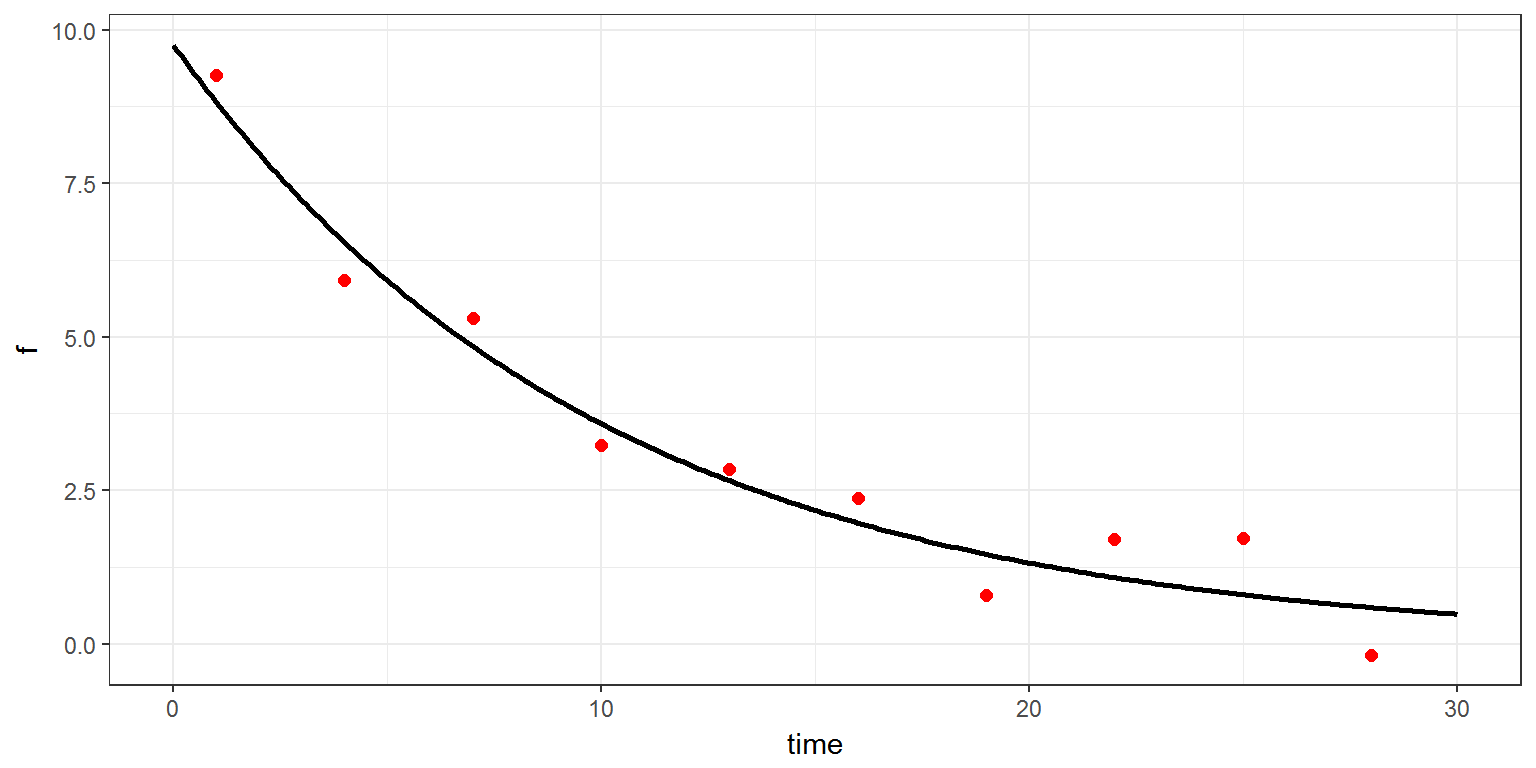Instead of only one individual, let us now simulate a group of 5 individuals with the same model. An individual parameter $$V_i$$ is drawn for each individual $$i=1,2,\ldots,5$$. The level of randomization is therefore ‘individual’. g <- list( size = 5, level = 'individual') res2 <- simulx(model = 'model/hierarchical1a.txt', parameter = p, output = list(V, f, y), group = g, settings = list(seed = 12345)) print(res2$parameter)
##   id         V
## 1  1 10.249302
## 2  2 12.908722
## 3  3  9.909589
## 4  4  8.703464
## 5  5 11.433969
print(ggplot() +
geom_line(data=res2$f, aes(x=time, y=f, colour=id),size=1) + geom_point(data=res2$y, aes(x=time, y=y, colour=id), size=2))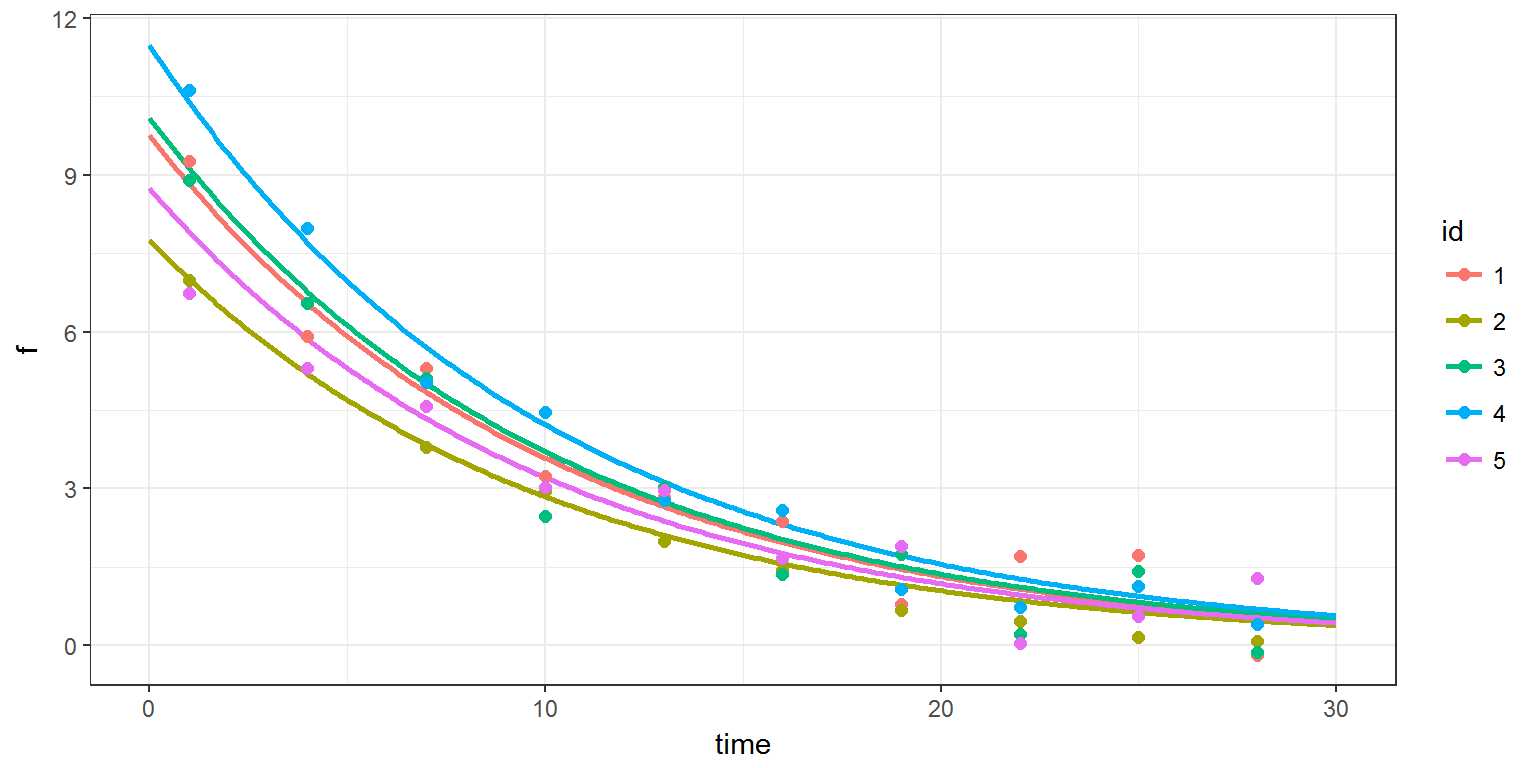If we now want to sample a single individual parameter $$V_1$$ for a single individual $$i=1$$, and 5 replicates of the longitudinal data for this individual, we must define the level of randomization as ‘longitudinal’.

g <- list( size  = 5,
level = 'longitudinal')

res3 <- simulx(model     = 'model/hierarchical1a.txt',
parameter = p,
output    = list(V, f, y),
group     = g,
settings  = list(seed = 12345))

print(res3$parameter) ## id V ## 1 1 10.2493 ## 2 2 10.2493 ## 3 3 10.2493 ## 4 4 10.2493 ## 5 5 10.2493 print(ggplot() + geom_line(data=res3$f, aes(x=time, y=f), colour="grey", size=1) +
geom_point(data=res3$y, aes(x=time, y=y, colour=id), size=2))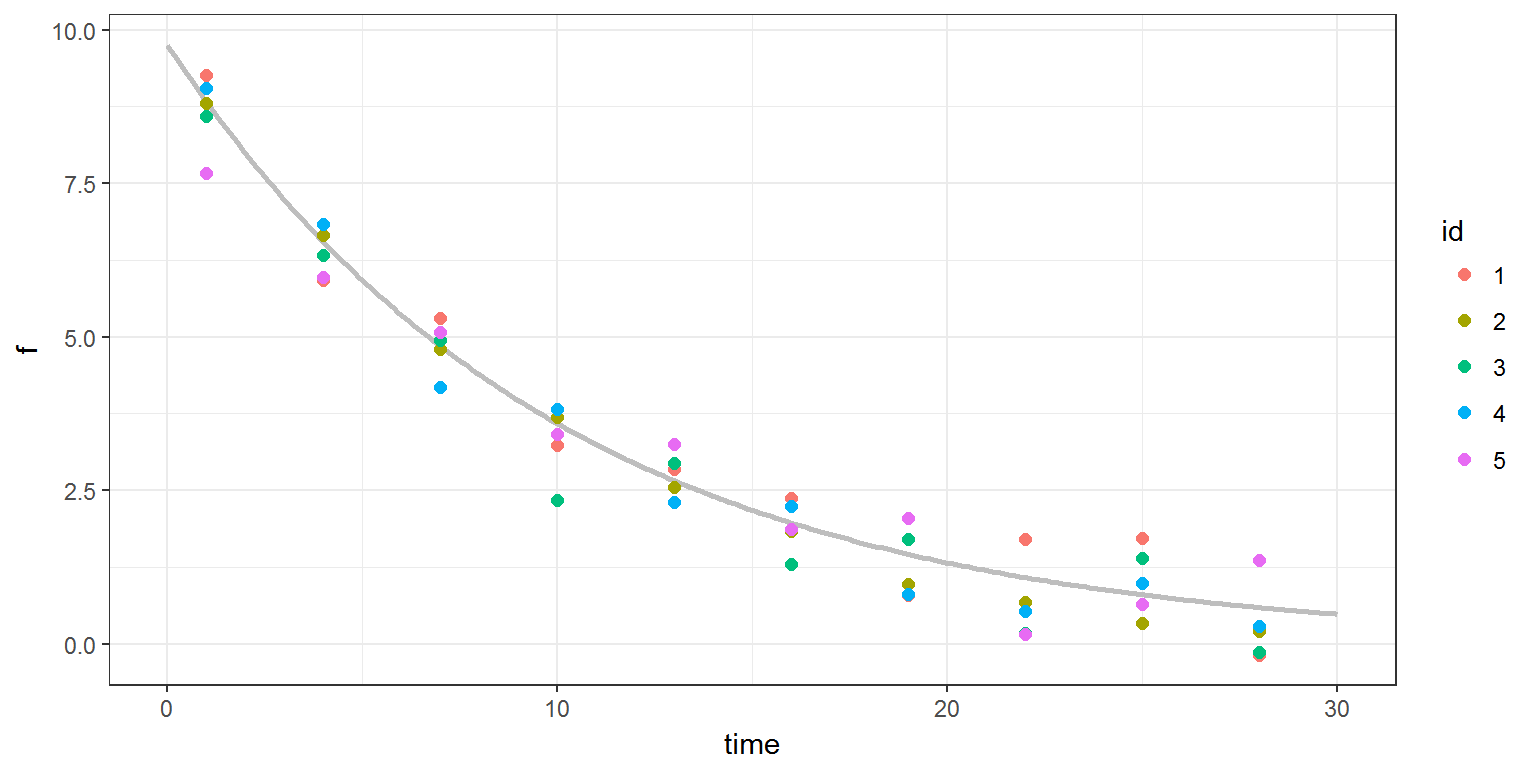It is possible to combine several levels of randomization. We may want, for example, to sample 2 individual parameters $$V_1$$ and $$V_2$$, and 3 replicates of the longitudinal data for each individual. g <- list( size = c(2,3), level = c('individual','longitudinal')) res4 <- simulx(model = 'model/hierarchical1a.txt', parameter = p, output = list(V, f, y), group = g, settings = list(seed = 12345)) print(res4$parameter)
##   id        V
## 1  1 10.24930
## 2  2 10.24930
## 3  3 10.24930
## 4  4 12.90872
## 5  5 12.90872
## 6  6 12.90872
print(ggplot() +
geom_line(data=res4$f, aes(x=time, y=f, colour=id), size=1) + geom_point(data=res4$y, aes(x=time, y=y, colour=id), size=2))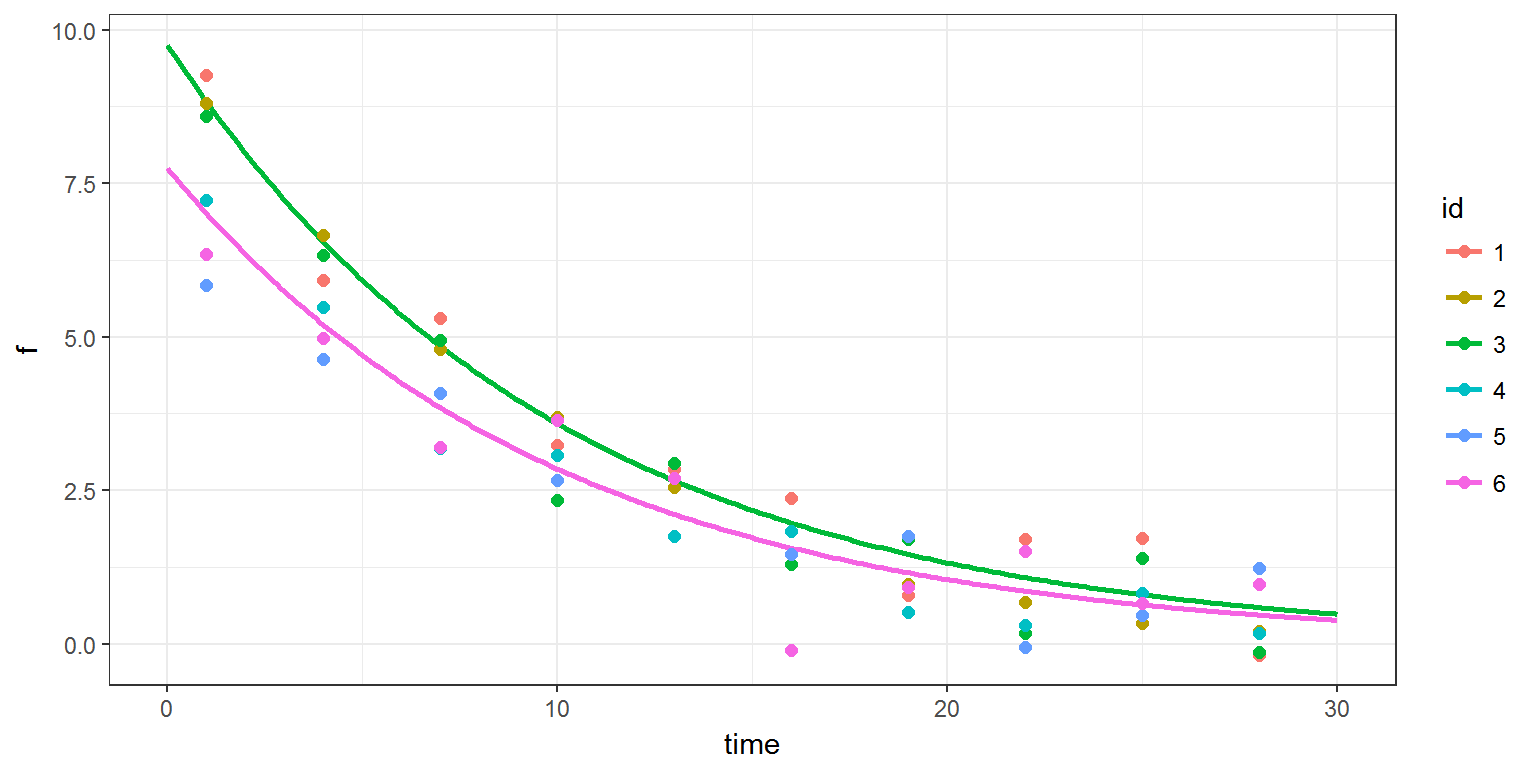## 2.2 Example 2

We still consider model (2) for the longitudinal data but $$V_i$$ is now function of the weight of individual $$i$$:

1. $\log(V_i) \iid {\cal N}\left(\log \left(V_{\rm pop} \left({w_i}/{w_{\rm pop}}\right)^{\beta}\right) \ ,\ \omega_V^2\right).$

This model is implemented in hierarchical1b.txt.

[LONGITUDINAL]
input = {V, k, a}
EQUATION:
f = 100/V*exp(-k*t)
DEFINITION:
y = {distribution = normal, prediction = f, sd = a}

[INDIVIDUAL]
input = {V_pop, omega_V, beta, w, w_pop}
EQUATION:
V_pred = V_pop*(w/w_pop)^beta
DEFINITION:
V = {distribution = lognormal, prediction = V_pred, sd = omega_V}


We therefore need to define the individual weight $$w_i$$ and the population weight $$w_{\rm pop}$$ as inputs of the model by adding them to the vector parameter.

p <- c(V_pop=10, omega_V=0.3, beta=1, w=75, w_pop=70, k=0.1, a=0.5)
f <- list(name='f', time=seq(0, 30, by=0.1))
y <- list(name='y', time=seq(1, 30, by=3))
V <- list(name='V')

g <- list( size  = 5,
level = 'individual')

res5 <- simulx(model     = 'model/hierarchical1b.txt',
parameter = p,
output    = list(V, f, y),
group     = g,
settings  = list(seed = 12345))

print(res5$parameter) ## id V ## 1 1 10.98140 ## 2 2 13.83077 ## 3 3 10.61742 ## 4 4 9.32514 ## 5 5 12.25068 print(ggplot() + geom_line(data=res5$f, aes(x=time, y=f, colour=id),size=1) +
geom_point(data=res5\$y, aes(x=time, y=y, colour=id), size=2))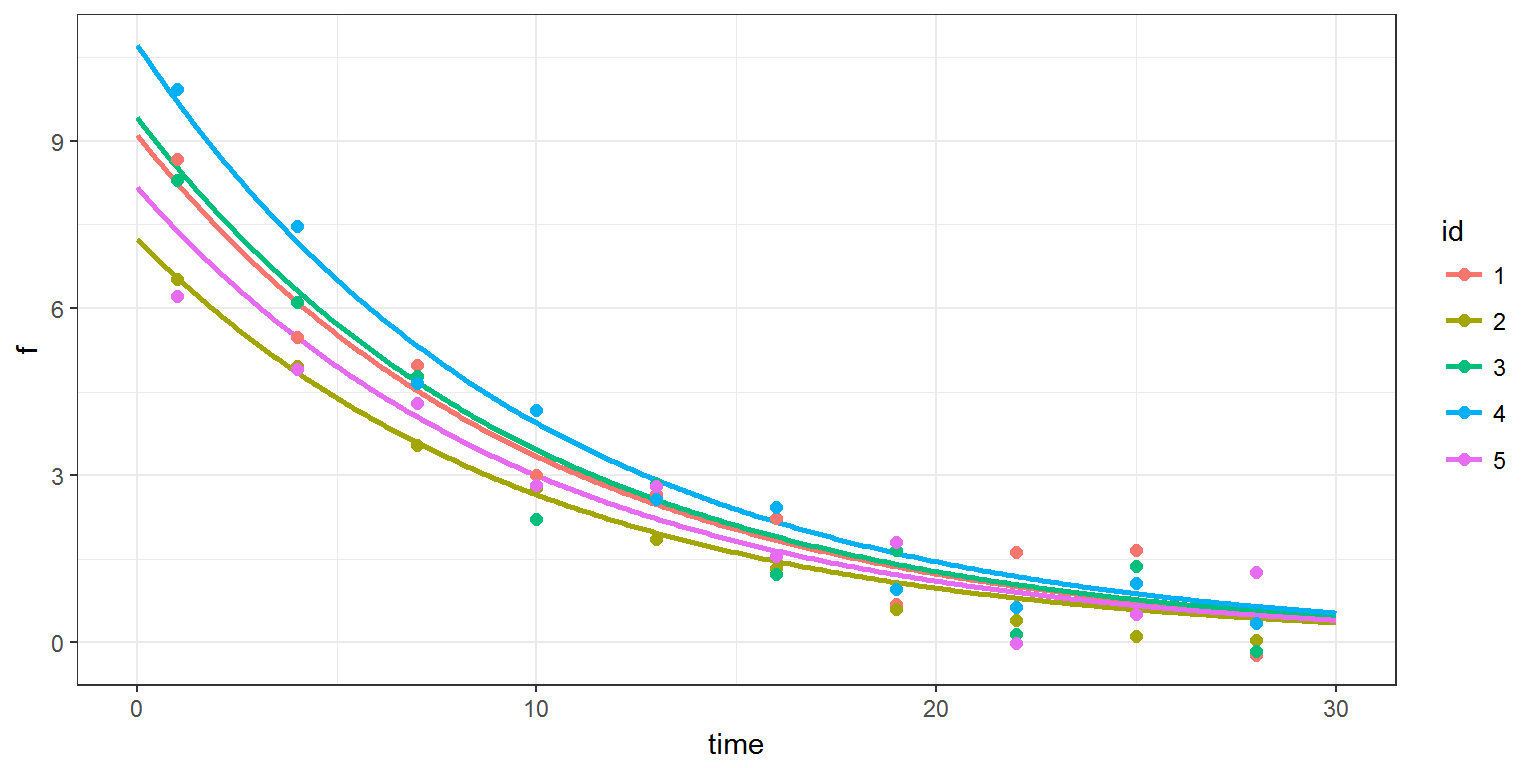Remark 1: The model for the individual parameter $$V_i$$ is a linear Gaussian model. Indeed, an equivalent mathematical representation of model (4) is the following one:

$\log(V_i) = \log(V_{\rm pop}) + \beta \log(w_i /w_{\rm pop}) + \eta_i$ where $$\eta_i \iid {\cal N}(0, \ ,\ \omega_V^2 )$$.

It is then possible to take advantage of this representation and implement the model for the individual parameters as a linear model. We could therefore replace the section [INDIVIDUAL] of hierarchical1b.txt with the following one:

[INDIVIDUAL]
input = {V_pop, omega_V, beta, w, w_pop}
EQUATION:
lw = log(w/w_pop)
DEFINITION:
V = {distribution=lognormal, reference=V_pop, covariate=lw, coefficient=beta, sd=omega_V}


Remark 2: The individual covariate $$w_i$$ is fixed and the same for all the individuals ($$w_i=75$$) in this examples. We will see in the next article how to simulate individual covariates. Individual covariates can also be provided in a data file.